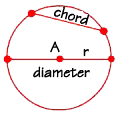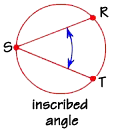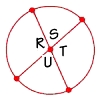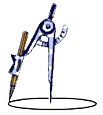Home    |    Teacher    |    Parents    |    Glossary    |    About UsLook around you and you'll find points, lines, planes, and angles of all sizes. Do you see any circular shapes among them? Perhaps the face of a clock, a lamp shade, a door knob, the base of a vase, or even a coffee mug? The rectangle is perhaps the "most seen" geometric figure, but the circle is a close second.

By definition, a circle is all points in the same plane that lie an equal distance from a given center point.

We might think of a dinner plate as a circle, but actually, it's not—it's a disk. The outer rim is a circle. A circle is just the points that lie on the curve itself. The points that lie inside the curve are called "interior points." A circle is named by its center point. This circle is circle "A."

The distance from the center point to a point on the circle is called the radius of the circle, shown in the diagram as r. The radius is a line segment with one endpoint on the circle and the other at the center of the circle.

The plural of radius is "radii." Two circles that have congruent radii, or radii that are the same size, are called congruent circles.

A diameter has both endpoints on the circle and must also contain the center of the circle. A diameter is twice the length of the radius.

Segments with both points on the circle are called chords. A diameter is a special type of chord, which passes through the center of the circle. As with any segments, two chords are congruent if their lengths are equal.

As you learned in an earlier lesson, a circle contains 360°. A portion of a circle is called an arc. Arcs are measured in degrees, like angles, and are classified in a similar way. There are minor arcs, major arcs, and semicircles. A minor arc measures between 0 and 180 degrees. A major arc is between 180 and 360 degrees. The semicircle is exactly 180°.

Arcs also have length. Imagine placing a string along a portion of a circle, then picking it up and stretching it out, and measuring it. That is arc length.

The sum of all the lengths of the non-overlapping arcs of a circle is called the circumference of that circle. This is the distance around the circle.

Two circles that have the same center are called concentric circles. The circles on a bull's-eye target are concentric.

When diameters intersect at the center of a circle, they form central angles. When you slice a round pizza, you usually slice along a diameter. If you slice a pizza into 8 congruent pieces, each section includes a central angle with a measure of 45°.

The measure of a central angle equals the measure of the arc that it "intercepts".

An inscribed angle is an angle with its vertex on the circle and its sides containing chords of the circle. Angle RST is an inscribed angle.Chords that intersect at any interior point form "interior angles." Angles r, s, t, and u are interior angles.This tool, called a compass, is used to construct circles. Obviously, this is different from a magnetic compass that is used to show direction. Like a protractor, a compass is a tool that every geometry student should have. It has many other uses in addition to circle construction, which you'll see in later lessons.Homework Help | Geometry | Geometry Building BlocksEmail this page to a friendSearch·  Words·  Coordinate geometry·  Pairs of lines·  Classifying angles·  Angles and     intersecting lines·  CirclesFirst Glance In Depth Examples WorkoutCircles